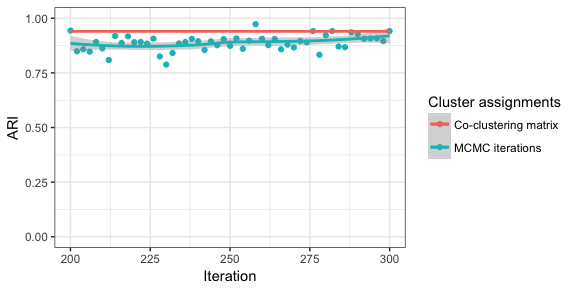# Context-dependent clustering with Clusternomics

#### 2017-03-14

This vignette demonstrates the usage of the clusternomics package for context-dependent clustering on a small simulated dataset.

The goal of context-dependendent clustering is to identify clusters in a set of related datasets. Clusternomics identifies both local clusters that exist at the level of individual datasets, and global clusters that appear across the datasets.

A typical application of the method is the task of cancer subtyping, where we analyse tumour samples. The individual datasets (contexts) are then various features of the tumour samples, such as gene expression data, DNA methylation measurements, miRNA expression etc. The assumption is that we have several measurements of different types describing the same set tumours. Each of the measurements then describes the tumour in a different context.

The clusternomics algorithm identifies

• clusters of measurements within individual datasets, we call these local clusters
• clusters of tumour samples that are informed by the local clusters, these are global clusters

## Warning: package 'ggplot2' was built under R version 3.3.2

## Simulated data

In the simulated scenario, we assume two contexts (datasets). In each context, the data are sampled from two clusters with the following distributions:

Cluster 1: $$x \sim \mathcal{N}\left((-1.5, -1.5)^T, (1,1)^T\right)$$

Cluster 2: $$x \sim \mathcal{N}\left((1.5, 1.5)^T, (1,1)^T\right)$$

Number of data points sampled from each cluster in each context is different:

Cluster 1 (context 2) Cluster 2 (context 2)
Cluster 1 (context 1) 50 10
Cluster 2 (context 1) 40 60

Using this setup, there are 160 data points in total. There are two local clusters within each context. At the same time, there are four clusters on the global level, that appear when we combine observations from the local contexts.

For the algorithm setup, we use set the number of clusters to a larger number than the original sampling distribution to examine how the algorithm behaves in this situation. We use set the numbers of clusters to 3 local clusters within each context, and up to 10 global clusters overall.

The inference in the model is done via MCMC sampling. We run the Gibbs sampler for 500 iterations, out of which 200 iterations are the burn-in iterations. Note that in larger non-simulated scenarios, the number of iterations should be larger.

## Algorithm setup

set.seed(1)

# Number of elements in each cluster, follows the table given above
groupCounts <- c(50, 10, 40, 60)
# Centers of clusters
means <- c(-1.5,1.5)
# Helper function to generate test data
testData <- generateTestData_2D(groupCounts, means)
datasets <- testData$data We look at the distribution of samples in the two contexts. The colours represent the four distinct clusters. qplot(datasets[][,1], datasets[][,2], col=factor(testData$groups)) +
geom_point(size=3) +
ggtitle("Context 1") + xlab("x") + ylab("y") +
scale_color_discrete(name="Cluster")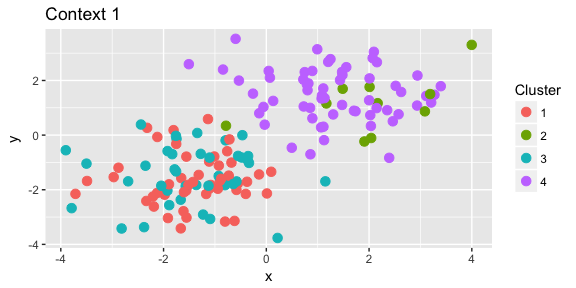qplot(datasets[][,1], datasets[][,2], col=factor(testData$groups)) + geom_point(size=3) + ggtitle("Context 2") + xlab("x") + ylab("y") + scale_color_discrete(name="Cluster")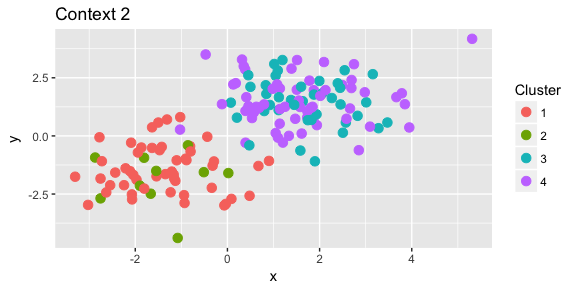Now we set-up the algorithm. We assume Gaussian distribution with diagonal covariance function in each context. As mentioned above, we pre-specify a larger number of clusters for the algorithm. The number of clusters that we use is not necessarily the number of clusters that the algorithm is going to use to model the data. It only serves as an upper limit on the number of clusters. # Setup of the algorithm dataDistributions <- 'diagNormal' # Pre-specify number of clusters clusterCounts <- list(global=10, context=c(3,3)) # Set number of iterations # The following is ONLY FOR SIMULATION PURPOSES # Use larger number of iterations for real-life data maxIter <- 300 burnin <- 200 lag <- 2 # Thinning of samples Finally, we can run the context-dependent clustering algorihm. # Run context-dependent clustering results <- contextCluster(datasets, clusterCounts, maxIter = maxIter, burnin = burnin, lag = lag, dataDistributions = 'diagNormal', verbose = F) # Extract resulting cluster assignments samples <- results$samples

# Extract global cluster assignments for each MCMC sample
clusters <-
laply(1:length(samples), function(i) samples[[i]]$Global)  ## Analysing clustering results We can check convergence of the model by looking at log likelihood values over the MCMC iterations: logliks <- results$logliks

qplot(1:maxIter, logliks) + geom_line() +
xlab("MCMC iterations") +
ylab("Log likelihood")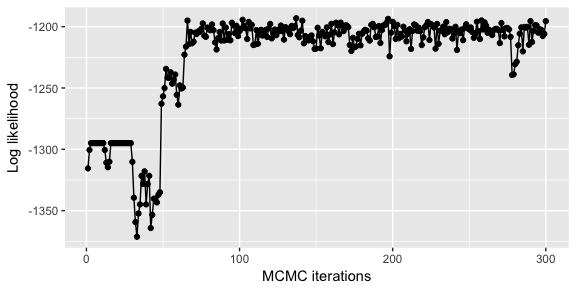### Choosing number of clusters

As part of the training, we also compute the Deviance Information Criterion (DIC). The DIC is a Bayesian model selection method that can be used to select the number of clusters. The DIC value is returned in results$DIC. Models that better fit the data will result in lower values of DIC than worse models. For example, if we fit a model with number of clusters that is too small, we get higher DIC value than for the original result. wrongClusterCounts <- list(global=2, context=c(2,1)) worseResults <- contextCluster(datasets, wrongClusterCounts, maxIter = maxIter, burnin = burnin, lag = lag, dataDistributions = 'diagNormal', verbose = F) print(paste('Original model has lower (better) DIC:', results$DIC))
##  "Original model has lower (better) DIC: 2437.7425595334"
print(paste('Worse model has higher (worse) DIC:', worseResults$DIC)) ##  "Worse model has higher (worse) DIC: 2595.24950967498" ### Posterior number of clusters We can also look at the number of global clusters that were identified in the datasets. The plot below shows the number of global clusters across MCMC samples. This is the number of actually occupied global clusters, which can be smaller than the number of global clusters specified when running the contextCluster function. cc <- numberOfClusters(clusters) qplot(seq(from=burnin, to = maxIter, by=lag), cc) + geom_line() + xlab("MCMC iterations") + ylab("Number of clusters")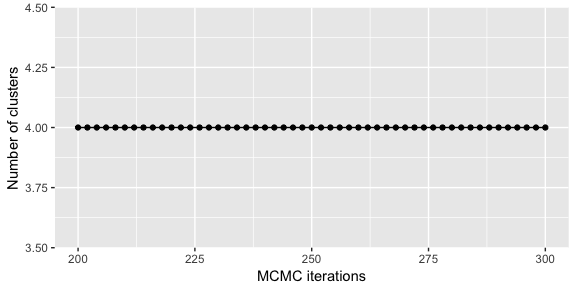### Sizes of clusters Here we look at the posterior sizes of the individual global clusters across the MCMC iterations and then we show a box plot with the estimated sizes. The labels of global clusters represent the corresponding combinations of local clusters. clusterLabels <- unique(clusters %>% as.vector) sizes <- matrix(nrow=nrow(clusters), ncol=length(clusterLabels)) for (ci in 1:length(clusterLabels)) { sizes[,ci] <- rowSums(clusters == clusterLabels[ci]) } sizes <- sizes %>% as.data.frame colnames(sizes) <- clusterLabels boxplot(sizes,xlab="Global combined clusters", ylab="Cluster size")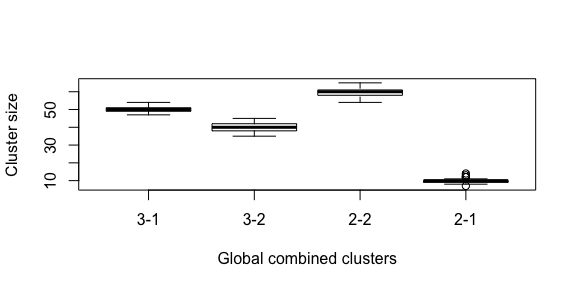## Obtaining global clusters There are several approaches to estimate hard cluster assignments from MCMC samples. If the MCMC chain converged to a stable results, it is possible to use one of the samples as the resulting cluster assignment. clusteringResult <- samples[[length(samples)]] ### Co-clustering matrix A more principled approach is to look at which data points were assigned into the same cluster across the MCMC samples. We can explore this using the posterior co-clustering matrix, which estimates the posterior probability that two samples belong to the same cluster. # Compute the co-clustering matrix from global cluster assignments coclust <- coclusteringMatrix(clusters) # Plot the co-clustering matrix as a heatmap require(gplots) mypalette <- colorRampPalette(rev(c('#d7191c','#fdae61','#ffffbf','#abd9e9','#4395d2')), space = "Lab")(100) h <- heatmap.2( coclust, col=mypalette, trace='none', dendrogram='row', labRow='', labCol='', key = TRUE, keysize = 1.5, density.info=c("none"), main="MCMC co-clustering matrix", scale = "none")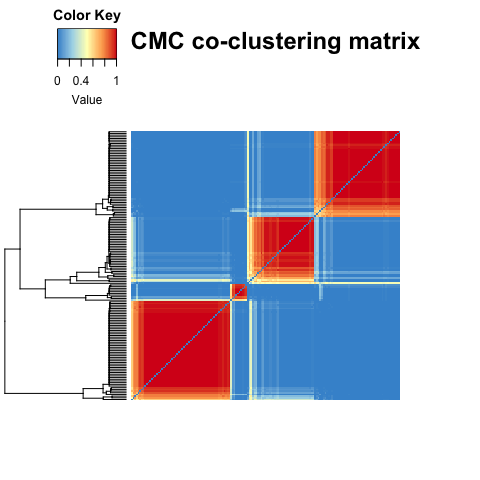We can then use the posterior co-clustering matrix to compute the resulting hard clustering using hierarchical clustering. Note that for this step, we need to specify the number of clusters that we want to obtain. This step should be guided by the posterior number of clusters estimated by the algorithm (see above). diag(coclust) <- 1 fit <- hclust(as.dist(1 - coclust)) hardAssignments <- cutree(fit, k=4) ### Adjusted Rand index Now we can check if the estimated global cluster assignments correspond to the true assignments that were used to generate the simulated dataset. We use the adjusted Rand index (ARI), which measures how well do two sets of assignments correspond to each other. The following plot shows the ARI across the MCMC iterations, and also the ARI of the result obtained from the co-clustering matrix. ARI equal to 1 represents complete agreement between the estimated assignments and the true clustering, ARI equal to 0 corresponds to random assignments. aris <- laply(1:nrow(clusters), function(i) mclust::adjustedRandIndex(clusters[i,], testData$groups)) %>%
as.data.frame
colnames(aris) <- "ARI"
aris$Iteration <- seq(from=burnin, to=maxIter, by=lag) coclustAri <- mclust::adjustedRandIndex(hardAssignments, testData$groups)
aris\$Coclust <- coclustAri

ggplot(aris, aes(x=Iteration, y=ARI, colour="MCMC iterations")) +
geom_point() +
ylim(0,1) +
geom_smooth(size=1) +
theme_bw() +
geom_line(aes(x=Iteration, y=Coclust, colour="Co-clustering matrix"), size=1) +
scale_colour_discrete(name="Cluster assignments")